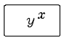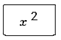## Exponents Assignment Help

Assignment Help: >> Introductory Mathematics - Exponents

Exponents:

Chapter exponents cover the addition, subtraction, multiplication & division of numbers with exponents.

APPLY one  of the arithmetic operations of addition, subtraction, multiplication & division using exponential numbers.

Calculator Usage, Special Keys

Exponent keyRaising a number to an exponent needs the yx  key to be pressed double.  First, the base number is entered and the yx  key is pressed; this enters the base number (y).  Further, the exponent number is pressed and the yx  key is pressed; this enters the exponent and tells the calculator to complete the calculation.  The calculator will display the value.

x squared keyPressing this key squares the displayed number.   This key will save time over using the yx  key.

 Basic Rules for Exponents Exponents Fractional Exponents Negative Exponents Zero Exponents#### Assured A++ Grade

Get guaranteed satisfaction & time on delivery in every assignment order you paid with us! We ensure premium quality solution document along with free turntin report!